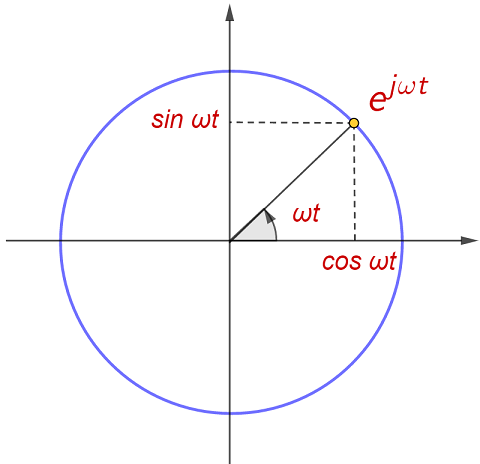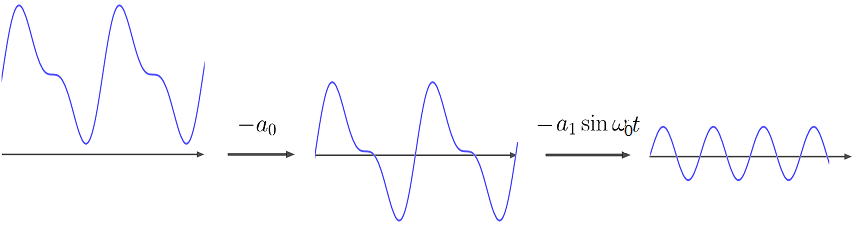2020-02-09
【信号与系统】02 - 傅里叶变换

# 1. 傅里叶级数

## 1.1 特征函数

上篇我们已经知道，LIT系统可以由单位冲激响应(h(t))完全表征，且(x(t))在系统的输出函数是(x(t)*h(t))。这个结论是分析LIT系统的基础理论，甚至我们可以认为，LIT系统至此已经被完全解析了。但不要忘记，解析信号系统的目的，最终是为了分析信号或系统的特性、设计特定系统以处理信号。所以下一步就是要建立分析信号或系统的方法，并搞清系统对信号产生的根本性影响。单位冲激响应(h(t))可视为系统的“固有信号”，所以接下来“信号”就是我们要面对的关键对象。

信号千变万化，一套完备的“特征”，一般要求它们互相独立、又能完整地表征对象。对待实变量函数，一种典型的思路是建立完备的“特征函数系”，特征函数之间有一定独立性，而任何函数可以被“特征系数”唯一表征。另外LIT是一个线性系统，在线性问题中有一个普遍而有效的思路，它非常类似于线性变换的特征向量理论。就独立性而言，我们希望“特征函数”(x(t))的系统输出有简单的格式(K(x(t))cdot x(t))。

为了找到特征函数，我们得回到系统的表征函数(int h(tau)x(t-tau)text{d}tau)中去。由其形式特点并联想到指数函数的特性，不难发现指数函数(e^{st})满足式（1），以后我们把这样的函数称为LIT系统的特征函数。其中(H(s))仅由系统和(s)决定，它是(e^{st})经过系统后的“特征系数”，也被称为系统的特征值。值得提醒的是，(s)是在复数域的。复指数函数的一般形式是(z^t)，但以(e)为低的复数表示更便于指数、导数等运算，故这里用(e^s)表示复数(z)。回顾复数的知识，(s=sigma jomega)，其中(e^sigmageqslant 0)是(z)的模，(omega)是(z)的辐角（顺时针为正向）。

[e^{st}to H(s)e^{st},,(sinBbb{C}),;;H(s)=int_{-infty}^{infty}h(tau)e^{-stau},text{d}tautag{1}]

## 1.2 三角基波

特征函数有很多，下面就要挑选合适的做“特征函数系”。当(sigmane 0)时，(e^{st})的范数(e^{sigma t})是无界的，使用起来比较棘手。这里先设(sigma=0)，纯虚数指数函数（式（2））将构成我们需要的特征函数系（也叫基波）。函数值(e^{jomega t})随(t)在单位圆上做圆周运动，(omega)是运动的角速度（弧度），运动的周期即为(2pi/|omega|)。教材里一般把(omega)称为基波频率，而真实的频率其实是(|omega|/2pi)，请注意区分。

[e^{text{j}omega t}=cosomega t jsinomega t,,(omegainBbb{R});;;T=dfrac{2pi}{|omega|}tag{2}](e^{jomega t})的实部、虚部都是正弦函数（这里不区分正余弦），正弦函数级数的性质已经被深入研究，用它们做基波是很方便的分析工具。傅里叶分析的早期研究对象，就是各种三角级数的收敛性。然而(e^{jomega t})才是三角函数更本质的形式，而且使用起来更加方便，所以今后我们都用复指数函数作为基波。复数域比实数域完备，所以复数运算更加方便自由，在纯数学上，不会因为一个概念“不够直观”而忽视它的价值。另外，系统的特征值(H(jomega))又被称为频率响应，它由冲激响应(h(t))所决定，后面将会看到它们的密切关系。

## 1.3 傅里叶级数

由于最早研究的函数分解就是三角函数级数，所以分解的对象也限定在了周期函数上。设函数(x(t))的周期为(T)，角速度为(omega_0)，傅里叶级数将它分解为基波频率为(komega_0,(kinBbb{Z}))的纯虚指数函数的级数（式（3）左）。为了求得系数(a_k)，可以利用({e^{jomega t}})对积分运算的“正交性”，验证可有式（3）右成立。式（3）就是傅里叶级数（FS）的完整表达式了，一般记作(x(t)overset{FS}leftrightarrow a_k)，其中(a_k)也称为FS的频谱系数

[x(t)=sum_{kinBbb{Z}}a_ke^{jkomega_0 t},;a_k=dfrac{1}{T}int_Tx(tau)e^{-jkomega_0tau},text{d}tautag{3}]

当然式（3）不是对所有周期函数都成立，狄利克雷条件给出了存在傅里叶级数的充分条件：（1）(x(t))绝对可积；（2）周期内只有有限个起伏；（3）周期内只有有限个不连续点。这个条件包含了非常大范围的函数，因此它有着很广泛的实用价值。值得提醒的是，在不连续点(t_0)处，傅里叶级数收敛于([x(t_0^-) x(t_0^ )]/2)，但在个别点的误差并不影响FS成为有力的分析工具。从表达式还能知道，相近的两个函数的频谱系数也是相近的，所以频谱系数具有一定“稳定性”。分解级数和积分式不一定存在，但如果一个式子存在，另一个式子也必然是存在的，且都具有唯一性。用带入证明会遇到根本性的困难，我们只能到傅里叶分析里找答案。

第一次面对FS的结论时，我们不禁想问：这个分解为什么会成立？它有没有更直观的解释？我想这样阐述（瞎说）FS的本质：函数就是一个随时间不断变化的量，这个“变化”可以从宏观到微观去依次去量化。就拿三角级数（4）来说，常数项(a_0)度量了(x(t))相对0值的平均变化，去除(a_0)后(a_1)继续度量每半边的平均变化（左右相等但符号相反，使用三角函数可统一系数），然后再继续度量半边的两个半边，以此类推。显然中心对称的函数都可以做基波，但唯有三角函数简单且有很好的分析性质。

[x(t)=a_0 a_1sinomega_0 t a_2sin2omega_0 ttag{4}]# 2. 傅里叶变换

## 2.1 傅里叶变换

傅里叶级数有着明显的局限性，最显然的就是它只适用于周期函数，而且如果把频谱系数作为函数的唯一表征，还必须限定在某个周期下。另一个缺陷不太明显但很重要，就是FS的频谱是不连续的，这限制了处理信号的范围。为了得到一般函数(x(t))的频谱，先以(t=0)为中心截取长度为(T)的片段，然后展开成周期函数(tilde{x}(t))。对它做FS可以得到式（5），其中({Ta_k})是函数(X(jomega))上的等间隔点。

Springboot SpringSecurity实现图片验证码登录问题

[Ta_k=X(jkomega_0),;;X(jomega)=int_Tx(t)e^{-jomega t},text{d}ttag{5}]随着(T)逐渐增大直至无穷，(tilde{x}(t))变成(x(t))，({Ta_k})也越发密集直至完全变成函数(X(jomega))（这当然不是严格的数学证明，但也不失为一个好的直观阐述）。从离散到连续的变化中，(omega_0)变成微分(text{d}omega)，(a_k)则变成了(dfrac{1}{2pi}X(jomega),text{d}omega)（因为(T=2pi/omega)）。最终（连加变成积分）(tilde{x}(t))的FS也变成了(x(t))的傅里叶变换（FT，式（6）），一般记作(x(t)overset{F}leftrightarrow X(jomega))，其中(X(jomega))还是称为频谱系数

[x(t)=dfrac{1}{2pi}int_{-infty}^{infty}X(jomega)e^{jomega t},text{d}omega;;;X(jomega)=int_{-infty}^{infty}x(t)e^{-jomega t},text{d}ttag{6}]

傅里叶变换也有对应的狄利克雷条件，只需把FS中的“周期内”改成“有限区间内”即可（以下把左式叫分解式、右式叫变换式）。狄利克雷条件是FT积分（处处）收敛的的充分非必要条件，在该条件下的分解式、变换式都是非奇异的。但在奇异函数的概念下，这些积分可以在更大的范围内“存在”，比如(delta(t))的FT是(1)，但分解式显然不收敛。所以这里要强调，傅里叶变换的存在性是比收敛性更宽泛的概念，数学上已经证明：分解式、变换式是同时存在的，且互相具有唯一性。

公式（6）说明了，函数和频谱系数是互相确定的，({e^{jomega t}})是一个完备的特征函数系。频谱系数可以完全表征一个函数，它一般被称为函数的频域特征，相对而言函数自身则是时域特征。时域、频域是分析信号或系统的两个角度，它们在不同的场景下有各自的长处。对于系统的冲激函数(h(t))，从式(1)可知，频率响应函数(H(jomega))就是(h(t))的傅里叶变换。也就是说，(h(t),H(jomega))分别是系统的时域、频域表征（后者也被称为系统函数），两者对系统分析都至关重要。

狄利克雷条件是FT收敛的充分而非必要条件，鉴于FS和FT的关系，下面来讨论怎样把FS纳入FT中去。其实FT的频谱系数(X(jomega))是不同基波的“密度”函数，(e^{jomega t})在分解中的“份量”是(dfrac{1}{2pi}X(jomega),text{d}omega)。反观FS的频谱系数(a_k)，(e^{jkomega_0})提供的“份量”就是(a_k)，它在FT中的“密度”应当是(2pi a_kdelta(omega-komega_0))。综合便有了FS的FT格式（式（7）），它其实就是周期函数的傅里叶变换。

[X(jomega)=sum_{kinBbb{Z}}2pi a_kdelta(omega-komega_0)tag{7}]

## 2.2 拉普拉斯变换

傅里叶变换的收敛性对函数有一定要求，比如函数一定要是有界的，这将限制对很多信号和系统的讨论。尤其在做系统的定性分析时，我们希望面对一个更大的系统空间进行系统设计。另一方面，LIT的特征函数(e^{st})中的(s)可取遍整个复数域，而FT的基波(e^{jomega t})仅仅是(s=sigma jomega)取虚轴而建立的函数系。为了研究用一般的(e^{st})为基波的分解，可以考虑在FT两边同时乘上函数(e^{sigma t})（式（8）），其中(sigma)是一个定值。

[x(t)e^{sigma t}=dfrac{1}{2pi}int_{-infty}^{infty}X(jomega)e^{(sigma jomega)t},text{d}omegatag{8}]

式（8）其实就是(x(t)e^{sigma t})在函数系({e^{(sigma jomega)t}})下的分解，由于(sigma)是一个定值，(s)取在某条跟虚轴平行的直线上。一般地，式（9）被称为拉普拉斯变换（LT），并计作(x(t)overset{L}{leftrightarrow}X(s))，它和FT显然有关系式（10）。(H(s))可以视为函数的(s)特征，它是对频域的扩充，在系统分析中也将起到更大的作用。还是得强调一下，虽然(X(s))的定义域可以是整个复数域，但在某个具体的拉普拉斯变换中，(s)仅在一条虚轴平行线上（(sigma)是定值）。课本中将逆变换写成了复数在曲线上的微分，我觉得对本课程没有意义。

[x(t)=dfrac{1}{2pi}int_{-infty}^{infty}X(s)e^{st},text{d}omega;;;X(s)=int_{-infty}^{infty}x(t)e^{-st},text{d}ttag{9}]

[x(t)overset{L}{leftrightarrow}X(sigma jomega);;Leftrightarrow;;x(t)e^{-sigma t}overset{F}{leftrightarrow}X(jomega)tag{10}]

对于一个函数(x(t))和固定的(sigma)，如果LT对所有的(omega)都收敛，那么称(x(t))的LT在(sigma)处收敛。那些收敛的(sigma)称为LT的收敛域（ROC），但要注意，收敛域外的某个具体(s)处，LT积分也可能收敛。课本上以(s)定义收敛域，其实并无本质区别，因为LT总是定义在整条虚轴平行线上的。如果(x(t))有限持续（(|t|>T)后为0），积分总是收敛的，它的ROC是整个复平面。如果(x(t))左边有限持续（右边信号），考察(x(t)e^{-sigma t})的绝对可积性，如果在(sigma_0)处绝对可积，则易证在(sigma>sigma_0)上都可积，从而ROC为右半平面。同样道理，左边信号的ROC就是左半平面。而一般的双边信号，可将其分割为左右两部分，结合刚才的结论可知，ROC是一个带状区域。

以上左/右平面、带状区域的边界是否收敛视情况而定，而且边界本身可能是不存在的（无穷大小），以下不再说明。冲激响应(h(t))的拉普拉斯变换(H(s))是系统的(s)域特征，它还是被称为系统函数，其ROC与系统性质有着一些关联。比如因果系统的冲击响应是一个右边信号，从而系统函数的ROC必定是右半平面。还有一个稳定系统的冲激响应是绝对可积的，从而它的傅里叶变换收敛，也就是说系统函数的ROC必须包含虚轴。